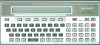##### Sharp PC-1500 : GHOST HOUSE game

"Ghost house" for Sharp PC-1500 +4KB.
Author : Akihiko Hatakeda, 2003
BASIC program.

Story: You have just arrived at the famous ghost house. Rumors say that there are amazing treasures in the house. Countless adventurers tried to get wealth and fame there, but no one came back...

How to play:
First time: DEF S
Next times: GOTO 10
To see top 30 scores: GOTO 6000

When you enter a new floor, you see 24 black squares representing 8 rooms (three black squares represent one room).
At each floor, there are three black gems, three monsters, and various other items hidden in the rooms.
You can explore 3 rooms per floor.
To explore a room, simply type its number (from 1 to 8).
After having explored 3 rooms, type another number to move to the next level.

You get score and experience by finding gems and killing monsters.
When the game starts, you have 500 hit points (HP) and monsters have 3 strength points (STR).
Unfound black gems at each floor are added to the monsters' strength. For example, if you do not find any of the three gems, STR increases by 3.
Finding the 3 black gems on a floor gives bonus and heals you.
Killing the 3 black monsters on a floor gives bonus and heals you.
The games goes on until your hit points fall below 0.
Things are easy at first because you start with many hit points - but monsters strength tend to get increase over time.

See attached scans for more explanations about monsters and gems.

BASIC program.

1 R=RND 24:IF S(R)>2THEN 1
2 S(R)=Q:RETURN
3 FOR X=15TO 25STEP 2:BEEP 1,X,20:NEXT X:CURSOR Z:RETURN
4 W=W-Q-1-KK:GOTO 970
5 CURSOR Z:GPRINT "11387D3811":RETURN
6 P=P+50*(Q+GA+1),K=K+.1:GOTO 970
7 P=P+80*M,K=K+.6:GOTO 970
10 PAUSE "** GHOST HOUSE 2003 **":RANDOM
20 PAUSE "** No.1";PT(I);" By ";PT\$(I):WAIT 0
30 P=0,S=500,M=1,0P=3,KO=3,W=1,HF=0,V=0,PR=0,RV=1,K=0,GA=0,WW=0
40 TT=-20,NO=I,AH=0,EN=3,AB=0,KA=0
130 PRINT "-- ";M;" FLOOR --":C=11111111
140 FOR Z=0TO 25:S(Z)=0:NEXT Z
150 Q=3:FOR Z=1TO 3:GOSUB 1
155 S(R-1)=S(R-1)+(S(R-1)<>3)
156 S(R+1)=S(R+1)+(S(R-1)<>3)
157 NEXT Z
160 IF M/3=INT (M/3)OR V>O OR NO>1LET Q=4:GOSUB 1
170 IF M>2AND RND 3=1LET Q=5:GOSUB 1:Q=8:GOSUB 1
180 IF M>4AND RND 3=1OR AH>0LET Q=6:GOSUB1
190 FOR Z=1TO 3+PR*9:Q=9:GOSUB 1:NEXT Z
200 IF W<-10LET Q=10:GOSUB 1
210 IF S>1000AND RND 3=1LET Q=11:GOSUB 1:Q=5:GOSUB 1
220 IF M>29AND RND 3=1LET Q=12:GOSUB 1
230 IF M>19AND RND 3=1LET Q=13:GOSUB 1
240 IF W>30LET Q=15:GOSUB 1
250 IF TT>19LET TT=0,Q=17:GOSUB 1
260 IF W/10=INT (W/10)LET Q=18:GOSUB 1
400 WAIT 0:FOR Z=1TO 24:CURSOR Z:PRINT CHR\$ 127;:BEEP 1:NEXT Z
410 KK=INT (K/4),Q=INT (M/8):IF M>111LET Q=13
450 A\$=INKEY\$ :A=VAL A\$,CC=O
470 IF A<1OR A>8OR A\$=""THEN 450
480 IF MID\$ (STR\$ (C),9-A,1)="2"THEN 450
490 C=C+10^(A-1),OP=OP-1:IF OP 500 FOR Z=A*3-2+(RV=-1)*2-AH*(RV=-1)TO A*3-2*(RV=-1)-AH*(RV=1)STEP RV
510 BEEP 1:CURSOR Z:GOTO STR\$ (S(Z))
600 "0"PRINT " ":IF S(Z-1)=9AND S(Z+1)=9THEN "14"
615 GOTO 970
620 "1"IF TT>15GOTO "0"
625 "2"GPRINT G\$(24+S(Z)):BEEP 3,15,12:GOTO 970
630 "3"GPRINT G\$(24):IF RV=-1THEN 705
635 BEEP 3,45,12:TT=TT+1,P=P+10*M,K=K+.05
636 IF CC=0AND RV=1AND S(Z-1)=3GOSUB 5
640 KO=KO-1:GOTO 970+(KO=0)*160
645 "4"GPRINT "70727E7270":FOR X=1TO 15:BEEP 2,X,30:NEXT X
650 IF S<10CURSOR Z:GPRINT "7C7D7F7D7C":S=S*500:FOR X=45TO 1STEP -5:BEEP 2,X,5:NEXT X
655 S=S+110+(10*KK)*OP,AB=1,OP=OP+V,V=0,NO=1:GOTO 6
660 "5"IF HF=2THEN 195
661 GPRINT "00201F2000":BEEP 1,45,20:BEEP 1,90,20:BEEP 1,45,20:HF=1
665 IF RND 3=1OR OP=0CURSOR Z:GPRINT "04207F2004": FOR X=255TO 5STEP -25:BEEP 1,X,10:NEXT X:HF=2
670 PR=0:GOTO 6
675 "6"GPRINT "7F4141411F":FOR X=1TO 2:BEEP 1,60,90:BEEP 1,150,30:NEXT X
680 W=W-3-RND(5+OP+KK*2),KA=Z,AH=0:GOTO 6
681 "7"GPRINT "007A7D1A00":OP=OP+1
682 FOR X=1TO 3:BEEP 1,220,5:BEEP 1,60,15:NEXT X:GOTO 6
685 "8"GPRINT "686F616F68":IF HF=0LET YY=10:GOTO 950
690 GOSUB 3:E=RND 6:GPRINT G\$(18+E):IF E=6THEN 635
695 P=P+50*M*E,K=K+.2,TT=TT+2:GOTO 4
700 "9"GPRINT G\$(Q):IF RV=-1THEN 635
705 IF HF=0LET YY=10:GOTO 950
710 GOSUB 3:GPRINT "2A0041002A":IF KA>0CURSOR KA:GPRINT "1A4742411F":GOTO 720
715 IF RND 6=1CURSOR Z:GPRINT "4411111744":AH=AH+(AH<2):GOTO 970
720 P=P+WW*10*(KK+1),K=K+.3,W=W-Q-1-KK,EN=EN-1:IF EN=0THEN 1175
725 IF RND 12 730 GOTO 970
735 "10"GPRINT "1E131F731E":FOR X=1TO 3:BEEP 1,15,20:BEEP 1,120,20:NEXT X:IF HF=0THEN 743
740 GOSUB 3:GPRINT "1018181810":P=P+100*M,K=K+1.5:GOTO 4
743 BEEP 1,20,380:W=ABS W:GOTO 970
745 "11"GPRINT "381C146438":IF HF=0LET YY=15:GOTO 950
746 IF AB=1BEEP 3,45,12:CURSOR Z:GPRINT "3A1C15643A":GOTO 7
750 GOSUB 3:GPRINT "356A186A35":V=V+(V<2):GOTO 970
760 "12"IF PR=1THEN "16"
761 GPRINT "4D7A08185F":BEEP 2,40,50:BEEP 1,40,350:IF HF=0LET PR=1,RV=1:GOTO 970
765 GOSUB 3:GPRINT "2D0244002B":P=P+300*Q,K=K+.8:GOTO 4
770 "13"IF PR=1THEN "16"
771 GRPINT "5F683F2B68":FOR X=100TO 50STEP -2:BEEP 1,X,5:NEXT X
780 IF HF>0GOSUB 3:GOTO STR\$(2+RND 5)
785 RV=RV*-1:GOTO 970
790 "14"CURSOR Z:GPRINT "341C7C7C34":BEEP 3,180,12:P=P+10*(Q+1),K=K+.05:IF HF=0THEN 970
795 E=RND 5:CURSOR Z:GPRINT G\$(13+E):FOR X=50TO 0STEP -5:BEEP 1,X,20:NEXT X
800 K=K+.8,P=P+100*M*E,TT=TT+3:GOTO 970
805 "15"GPRINT "31691A6C34"
810 FOR X=180TO 150STEP -6:BEEP 1,X,10:BEEP 1,X-90,10:BEEP 1,X-90,10:BEEP 1,X-90,10:NEXT X
815 IF HF>0AND K>10GOSUB 3:GPRINT "30621F6230":P=P+WW*200,K=K+2:GOTO 4
820 S=INT((S-WW*W+KK)/10):GOTO 970+(S<1)*200
840 "16"GPRINT "431E071E43"
850 FOR X=40TO 20STEP -10:FOR XX=20TO 40STEP 10:BEEP 1,X,10:BEEP 1,XX,10:NEXT XX:NEXT X
855 IF HF>0AND K>6GOSUB 3:GPRINT "2B0241022B":P=P+WW*100:K=K+1.8:GOTO 4
860 YY=30:GOTO 950
880 "17"GPRINT "4278097842":FOR X=120TO 100STEP -4:BEEP 1,X,10:BEEP 1,X+15,10:BEEP 1,X-15,10:NEXT X
890 P=P+S*10*KK,K=K+1.3:GOTO 970
895 "18"IF HF>0THEN "7"
900 GPRINT "1F217E3F1F":BEEP 1,255,20:BEEP 1,155,20:BEEP 1,55,20
910 IF AB=1BEEP 3,45,12:CURSOR Z:GPRINT "3A4871783A":GOTO 7
920 NO=NO+1:GOTO 970
950 BEEP 3,120,60:S=S-RND(YY+Q-KK)*W:IF S<1THEN 1170
970 CC=CC+1:NEXT Z:IF S(Z+1)=3AND S(Z)=3AND RV=1GOSUB 5
975 GOTO 450
990 CLS:WAIT 96:BEEP 5:PRINT "** Go to next FLOOR **":KA=0,AB=0
1010 W=W+KO*NO,WW=WW+KO*NO,HF=HF-(HF>0),M=M+1,0P=3-V,KO=3,EN=(PR=0)*3,CC=0
1030 WAIT 48:GPRINT "7C107C007C141C";:PRINT S
1050 CURSOR 9:GPRINT "5C547400047C04007C345C00";:PRINT W 1090 CURSOR 15:PRINT P;:GPRINT "7C141C00047C04005C54740040":GOTO 130
1130 WAIT 96:CLS:BEEP 5,20,10:PRINT "** TAKE ALL GEMS! **";:IF RV=-1CURSOR 16:PRINT "?"
1150 P=P+100*M*(KK+1),S=S+100+INT(S*((KK+3)/10)),W=W-Q-4-KK,K=K+.5,GA=GA+1
1160 AH=0,V=0,PR=0,RV=1,NO=1:GOTO 990
1170 WAIT 96:BEEP 4,100,120:PRINT "** R.I.P. **";P:Z=1:GOTO 5010
1175 WAIT 96:BEEP 5,20,10:PRINT "** KILL ALL GHOSTS! **":GOTO 1150
1180 "S"DIM S(25),G\$(26)*10,PT(31),PT\$(31)*5:RESTORE 1230
1190 FOR Z=0TO 26:READ G\$(Z):NEXT Z:GOTO 10
1230 DATA "407E027E40","013F203F01","1070405E72","2871517926","3E6B6F6B2E"
1240 DATA "40701F1F1F","32797E7932","2659295926","19260C2619","5C6E3E6E5C"
1250 DATA "4C6B1F6B4C","2532706522","5A2703275A","5A6F3D6F5A"
1260 DATA "2670232026","7679732076","7650535076","0600030006","240E5F0E24"
1270 DATA "2250515022","3A4445443A","0238453802","3248454832","1228492812"
1275 DATA "12387D3812","0820742008","0C2076200C"
5010 IF P 5020 IF P 5040 PRINT "You are No.";Z:INPUT "Name? ";N\$
5050 FOR X=31TO ZSTEP -1:PT(X)=PT(X-1),PT\$(X)=PT\$(X-1):NEXT X:PT(Z)=P,PT\$(Z)=N\$
5090 WAIT :PRINT "** GAME OVER **";P
6000 FOR Z=1TO 30:PRINT "No.";Z;" ";PT(Z);" ";PT\$(Z):NEXT Z